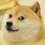# Linear Mean Function

I've been looking at functions $$f:\mathbb{R}^n \to \mathbb{R}$$ which necessarily satisfy the following 3 properties. Given $$a_1, a_2, \dots a_n \in \mathbb{R}^+$$

$\begin{array} { l l } 1. & f(x_1 + c, x_2 + c, \dots , x_n + c) = f(x_1, x_2, \dots , x_n) + c \\ 2. & f(cx_1, cx_2, \dots , cx_n) = cf(x_1, x_2, \dots, x_n) \\ 3. & \sum_{i=1}^n a_i x_i = 0 \Leftrightarrow f(x_1, x_2, \dots, x_3) = 0 \end{array}$

I believe that the the only function that satisfies this is $f(x_1,x_2, \dots, x_n) = \frac{\sum_{i=1}^n a_i x_i}{\sum_{i=1}^n a_i}$

I have the proof too while I'll write up later. I'm interested in all of your interpretations of this.

What happens if we only have 2 out of these 3 conditions?Note by Josh Banister
4 years, 8 months ago

This discussion board is a place to discuss our Daily Challenges and the math and science related to those challenges. Explanations are more than just a solution — they should explain the steps and thinking strategies that you used to obtain the solution. Comments should further the discussion of math and science.

When posting on Brilliant:

• Use the emojis to react to an explanation, whether you're congratulating a job well done , or just really confused .
• Ask specific questions about the challenge or the steps in somebody's explanation. Well-posed questions can add a lot to the discussion, but posting "I don't understand!" doesn't help anyone.
• Try to contribute something new to the discussion, whether it is an extension, generalization or other idea related to the challenge.

MarkdownAppears as
*italics* or _italics_ italics
**bold** or __bold__ bold
- bulleted- list
• bulleted
• list
1. numbered2. list
1. numbered
2. list
Note: you must add a full line of space before and after lists for them to show up correctly
paragraph 1paragraph 2

paragraph 1

paragraph 2

[example link](https://brilliant.org)example link
> This is a quote
This is a quote
    # I indented these lines
# 4 spaces, and now they show
# up as a code block.

print "hello world"
# I indented these lines
# 4 spaces, and now they show
# up as a code block.

print "hello world"
MathAppears as
Remember to wrap math in $$ ... $$ or $ ... $ to ensure proper formatting.
2 \times 3 $2 \times 3$
2^{34} $2^{34}$
a_{i-1} $a_{i-1}$
\frac{2}{3} $\frac{2}{3}$
\sqrt{2} $\sqrt{2}$
\sum_{i=1}^3 $\sum_{i=1}^3$
\sin \theta $\sin \theta$
\boxed{123} $\boxed{123}$

Sort by:

Let $x_1$, $x_2$, $\dots$, $x_n$ be given real numbers. We seek a value of $c$ so that $a_1 (x_1 + c) + a_2 (x_2 + c) + \dots + a_n (x_n + c) = 0.$ Solving for $c$, we get $c = -\frac{a_1 x_1 + a_2 x_2 + \dots + x_n x_n}{a_1 + a_2 + \dots + a_n}.$

From the third property, $f(x_1 + c, x_2 + c, \dots, x_n + c) = 0$, so by the first property, $f(x_1, x_2, \dots, x_n) = -c = \frac{a_1 x_1 + a_2 x_2 + \dots + x_n x_n}{a_1 + a_2 + \dots + a_n}.$ Note that the second property is never used.

- 4 years, 8 months ago

The idea behind the second property is what happens if the third one was never there? (What happens now if we only assume the first 2 ideas. Hence the linear part of the title)

- 4 years, 8 months ago

That's very interesting! I believe there is a geometric interpretation of the result, as the (normalized) distance of the vector x projected onto a.

Staff - 4 years, 8 months ago